# RFEM – Online Manual

Online manuals, introductory examples, tutorials, and other documentation.

# 7.4 Starting the Calculation

There are several ways to start the calculation. It is recommended to perform a short plausibility check of input data beforehand (see Chapter 7.1.1).

Calculate All

To start the corresponding function, use the menu item

• Calculate → Calculate All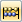or the corresponding toolbar button.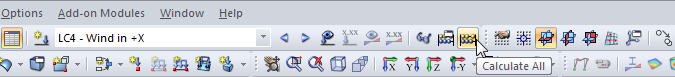Figure 7.30 [Calculate All] button

This command starts the calculation of all load cases, load combinations, and result combinations, as well as all add-on modules where input data is available.

The [Calculate All] function should be used with care:

• Many load cases cannot occur on their own. Wind loads, for example, always act together with self-weight. For structures with failing supports for tension, instabilities may occur during the batch calculation of all single load cases.
• If many load combinations and module design cases are available, RFEM may need a lot of computing time.
Calculating selected load cases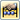To open the dialog box for selecting the load cases relevant for calculation, use the menu option

• Calculate → Calculate All.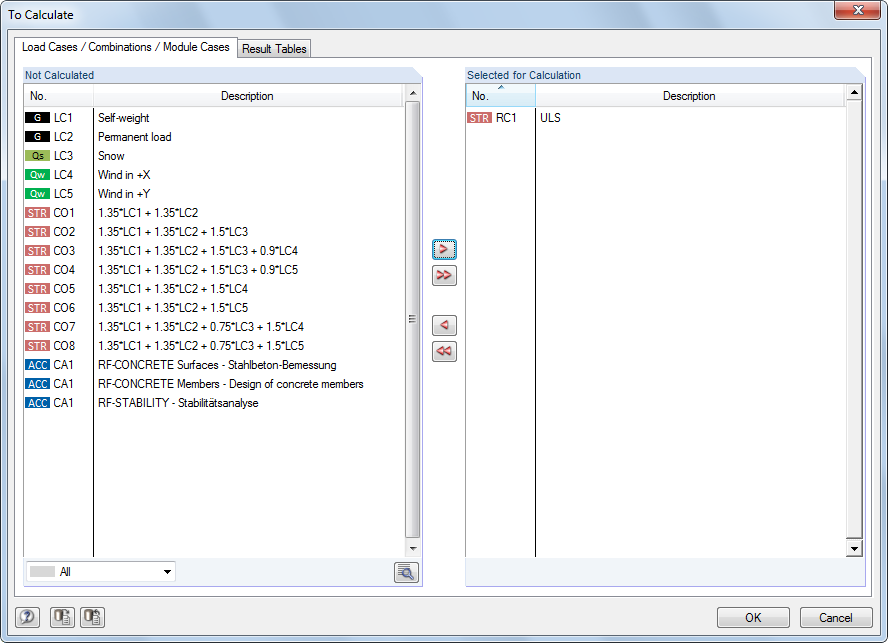Figure 7.31 To Calculate dialog box

In the Not Calculated dialog section on the left, RFEM lists all load cases, load combinations, result combinations, and analysis cases of add-on modules for which no results exist. Use the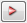button to transfer the selected entries into the Selected for Calculation list. You can also double-click the items. To transfer the entire list to the right, use the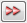button.

If result combinations or add-on module cases that require results from load cases are selected, the relevant load cases are automatically included in the calculation.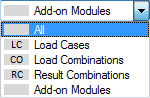The list can be sorted according to certain criteria with the filter options shown on the left.

The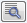button opens the Calculation Parameters dialog box (see Chapter 7.3) where you can check and adjust settings for the calculation.

The Result Tables tab of the To Calculate dialog box controls which tables are available after calculation.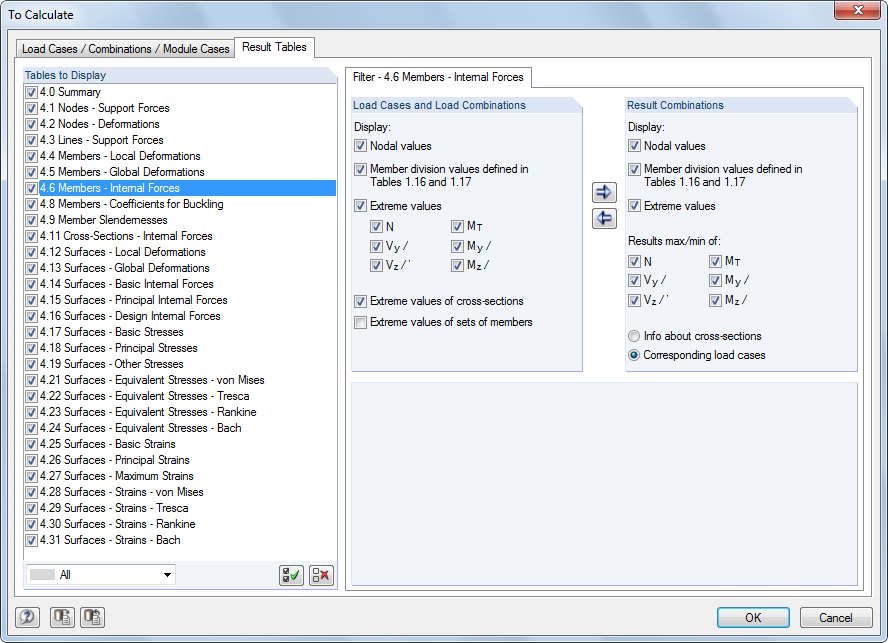Figure 7.32 To Calculate dialog box, Result Tables tab

Additional filter options are available for some results tables. They are presented in Chapter 8 together with the respective output tables (see Figure 8.14, for example).

Calculating current load case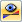It is possible to directly start the calculation of an individual load case: Select the load case, load combination, or result combination in the toolbar list, then click the [Show Results] button.Figure 7.33 Calculating the load case directly by using the [Show Results] button

The calculation can be started after a message has been displayed that says that no results have been found.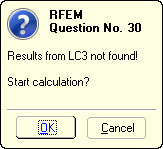Figure 7.34 Query before calculation
Calculating selected results

The Calculate toolbar menu provides additional options for selecting results you want to calculate:

• RFEM results only
• Modules results only
• All results of all open models
• RFEM results only of all open models
• Modules results only of all open models

The calculation starts immediately after calling the corresponding function.

Calculation process

The calculation process is shown in the FE-Calculation window. In a diagram of the convergence process, you can observe the shapes of the maximum displacements in addition to the passed calculation steps.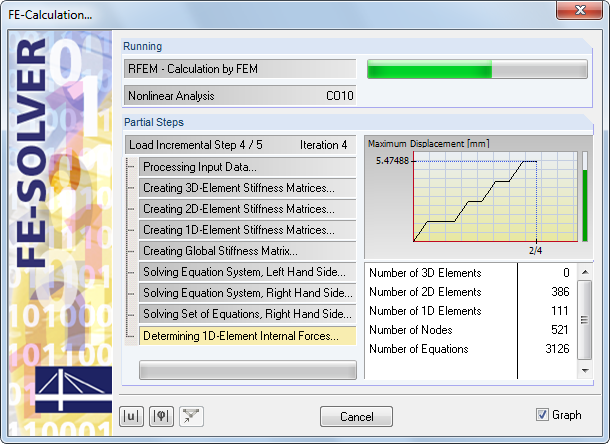Figure 7.35 Calculation process

The green or red vertical bars on the right in the window visualize the convergence behavior during the calculation: Each load increment takes up a part of the column; for example, 4/5 in the figure above is the fourth of five load increments. When the bar is green, deformations are in a tolerable zone, whereas a red bar symbolizes oversized displacements or rotations (≥ 0.1 rad).